List of notes taken while studying for my Combinatorics midterm.

```Studying for Combinatorics Midterm

Perfect covers

For covering an m by n board, we know that a perfect cover is possible if and only if a trivial perfect cover is possible.

That means that if a perfect cover is possible, we know a trivial cover is possible as well.

Officers

For dealing with organizing so that there are no officers of the same rank or regiment in either the same column or row, you can construct two different arrays where each of them follow the rule, and then just put them together.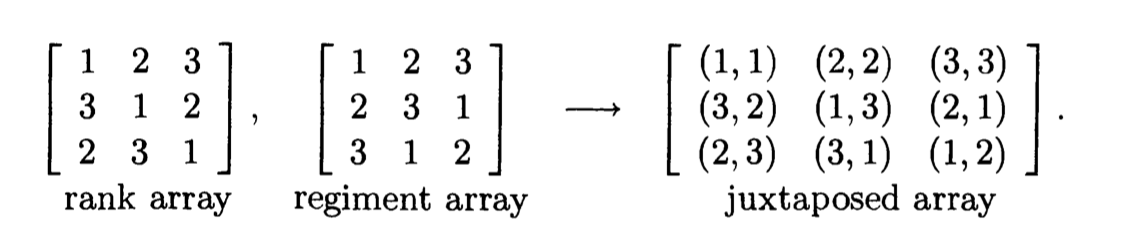A pair of arrays is orthogonal, where all possible combinations result (1,1), (1,2), etc.

Circular Permutations

I don't really see how this works, but the equation is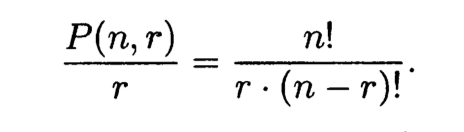More intuitively, say we have to permute the letters A-E at a circular table. We do the typical equation, and then divide by the number of elements. So apparently the seats are non-unique, and so if you had one orientation, and rotated that orientation to the right, that would be the same ordering in this example. So it now makes sense why you need to divide by 6. If the seats mattered, you shouldn't have had to divide.

Problems on the test:
Out of 30 points. First 4 are worth 28 points.

1. Cards  (exactly)
Imagine you are given 13 cards, and say that you are to find how many pure combinations of sequences of 3 and sequences of 2 (pure meaning 123-45 is not allowed) are possible. First, imagine three slots followed by two slots (U U U - U U) and now fill the first three with 123. We see that 45 are not possible, so only 5,6-12,13 are possible. This is 8 possibilities. Then, we increment the starting sequence of 123 to be 234 which gives up 7 possibilities. We see that we are adding 8+7+6… But before we apply the n(n+1)/2 formula, we need to remember that the abstraction of UUU-UU was just to help us figure out a partial answer, and that we need to also take into account the (distinct) UU-UUU possibilities. With a little investigation, we find that it is also 8+7+6 and so we have 2*(n(n+1)/2) which is n*n+1 = 72.

2. R(n,r) & inclusion exclusion (exactly)
Say you have  3A's 4B's and 5C's and you are to find all the ways you can take ten items from them. We have to use the formula (r+k-1) choose (k-1) where r=size of sample and k=how many types.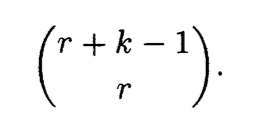So r would be 10 and k would be 3. So we would be doing (12) choose (2) = 66. This is how many ways you could pick up 10 items from 3 types of items, each of which are in unlimited supply. The problem is that you counted combinations where you took more than the allowed number of A’s, B’s, and C’s. So let’s first subtract all the times we took too many A’s, we do this with the same formula but we’re imagining that we’ve already selected an invalid number of A’s, which means we imagine we’ve selected 4 A’s, so we look at all the ways we could have put the three types in the remaining 6 spots, so r=6 and k=3 so (8 choose 6) = 28, and we do the same for the other two (B is r=5 and k=3 so (7 choose 5)=21) and then you subtract all of these from 66 but you realize that you overcounted because there could have been times when you had four or more A’s _and_ more than five B’s, so we have to re-add when you have 4 A’s+5 B’s so 9 fixed and one varying which using the equation is (1+3-1 choose 1) = (3 choose 1) = 3 which makes sense because you have one spot varying for all 3. Then you subtract the triple intersection and then you’re done.

3. Newton's binomial  (for pnts?)
Could just be as simple as using the formula: (x+y)^n = sigma from k=0 to n (n choose k) x^k 	y^n-k.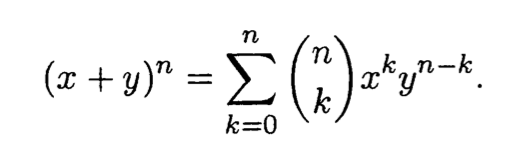If it’s not just plug and play, and it’s using newton’s binomial to prove something, look at the case where y=1 and you have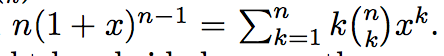, and then maybe you could use this to prove something. Keep in mind you may not be plugging in 1, but you could plug in two.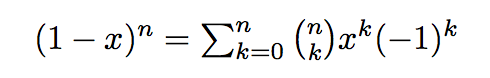and adding that with the original gives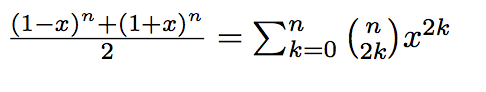. You use this by plugging in the x value.

4. Recurrence relation (very simple, rabbits or something similar).

Perfect covers: If we're dealing with perfect covers with some restrictions, say n by 2, covering with black and red dominoes where no two red dominoes can be horizontally on top of each other. Well, we can simplify this by looking at the n by 1 case (looking at one of the columns) because we know that one column actually reflects how the other is setup. Then, let's say we have some perfect covering of h_n, and let's look at the h_n+1 case. Well, for the new spot we have a lot of options. We could put a black domino sideways which would give us h_n possibilities, we could also put a black one vertical which would be h_n-1 possibilities. We could put a red one vertically, which would be h_n-1 possibilities because there are no restrictions on vertical red dominoes, or we could put it horizontally. If we put it horizontally, we couldn't take the h_n count because this count includes possibilities where there is already a horizontal red at the end, so we must take the case where we have a black domino at the end which would be h_n-1. So we get h_n+1 = h_n + 3h_n-1. or h_n=h_n-1 + 3h_n-2.

Solving recurrence relations: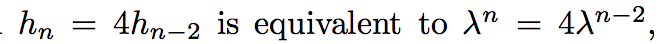then divide by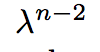(or the lowest n term), to get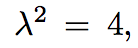. You have to solve for lambda, which you can use the quadratic formula for.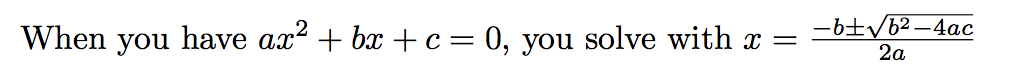(where x is lambda). So when you get the roots, you should write h_n= C1(root one)^n + C_2(root two)^n. Then you have to determine the initial conditions based on looking at the problem, and plugging in “n” and solving for C’s.

5. Composing (top secret)
Compositions may mean "how many different ways can you write the number 5" which could be 1+1+1+1+1 or 2+3 or 1+1+3 etc. Or, it could be very similar but just with a slightly different application?

Important formulas: Choosing r from n is:

n!
------------------------
r!  (n-r)!

So for 15 choose 4 it's

15!
------------------------
4! 11!

```